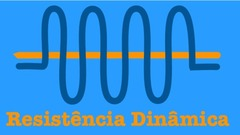RESISTÊNCIA DINÂMICA - Hölder continuity of the Lyapunov exponent for linear cocycles over hyperbolic dynamical systemsDynamical Resistance Day

 Hölder continuity of the Lyapunov exponent for linear cocycles over hyperbolic dynamical systems17 de Abril | 11h00min | Pedro Duarte (Univ. Lisboa)

Title: Hölder continuity of the Lyapunov exponent for linear cocycles over hyperbolic dynamical systems (video)

In this talk we explain how to derive Holder continuity of the Lyapunov exponent
for fiber bunched GL(d,R)-linear cocycles over hyperbolic maps. A generic pinching and twisting assumption is required. In other words, if the cocycle is assumed to satisfy the simplicity criterion of Avila and Viana  then all Lyapunov exponents depend Holder continuously on the cocycle matrix valued function.
To set the context, in a recent paper , Backes, Brown and Butler proved the continuity of the Lyapunov exponent for fiber bunched GL(2,R)-linear cocycles over hyperbolic maps, without any generic assumption on the cocycle, thus proving a conjecture of Viana and at the same time generalizing a theorem of Bocker-Neto and Viana  about the continuity of the Lyapunov exponent for locally constant random GL(2,R)-linear cocycles. The Holder quantitative version of  (with a generic assumption and for arbitrary dimensions) was established by E. Le Page many years before in . The corresponding higher dimensional generalizations of  and  are respectively a work under preparation  by Avila, Eskin and Viana and something more difficult yet to be done.
Joint work with Silvius Klein e Mauricio Poletti.

 A. Avila, A. Eskin, and M. Viana, Continuity of Lyapunov exponents for products random matrices, In preparation.
 A. Avila and M. Viana, Simplicity of Lyapunov spectra: a sufficient criterion, Port. Math. 64 (2007), 311–376.
 L. Backes, A. Brown, and C. Butler, Continuity of Lyapunov exponents for cocycles with invariant holonomies, Preprint http://arxiv.org/pdf/1507.08978v2.pdf.
 C. Bocker-Neto and M. Viana, Continuity of Lyapunov exponents for random 2d matrices, preprint (2010), 1–38.
 E. Le Page, Régularité du plus grand exposant caractéristique des produits de matrices aléatoires indépendantes et applications, Annales de l’institut Henri Poincaré (B) Probabilités et Statistiques 25 (1989), no. 2, 109–142.